## PICO-8

This PICO-8 guide helps you find the code or commands you need, based on what you want to do. It also helps explain the basics of programming, how to use functions, and provides many examples. Whether you are new to PICO-8 or not, this guide is a nice reference companion while making games in PICO-8.

If you already know the code, and want to search by that, try these:

`Wiki Reference`

`Cheatsheet by Neko250`

# PICO-8 Guide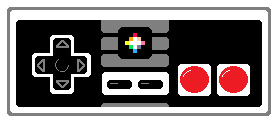• ## PICO-8 Basics

• ### Main Screen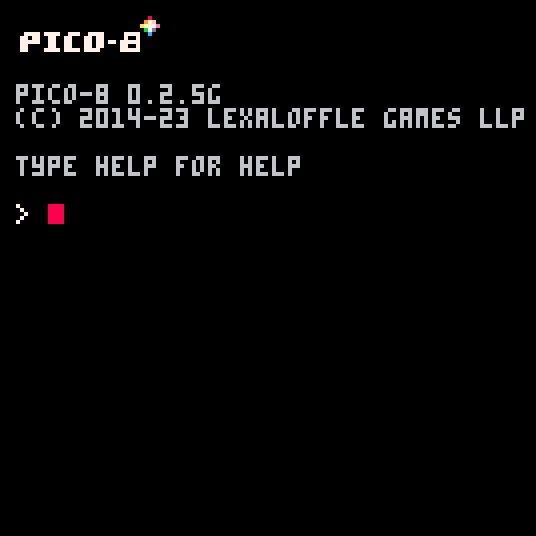This is what you will see every time you start up PICO-8. It is a fun retro style command line like the original home computers. You have to type in commands to navigate your folders and load your games.

To begin editing a blank game, just press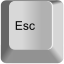• ### Game Editors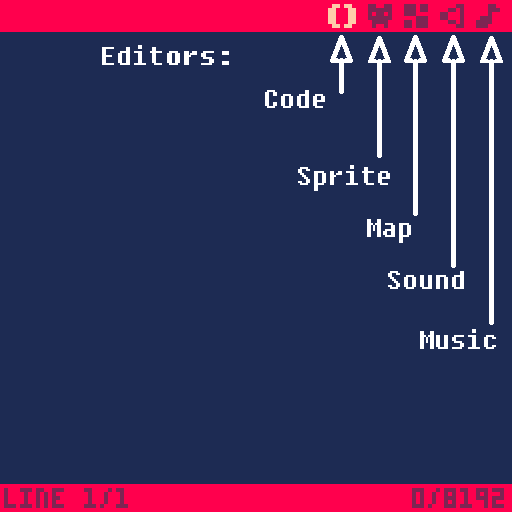• ### Code Editor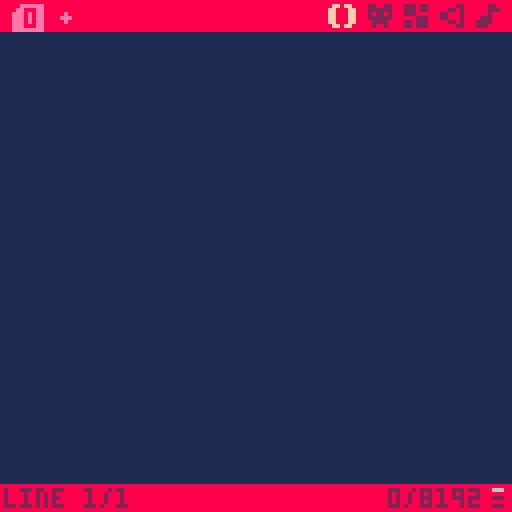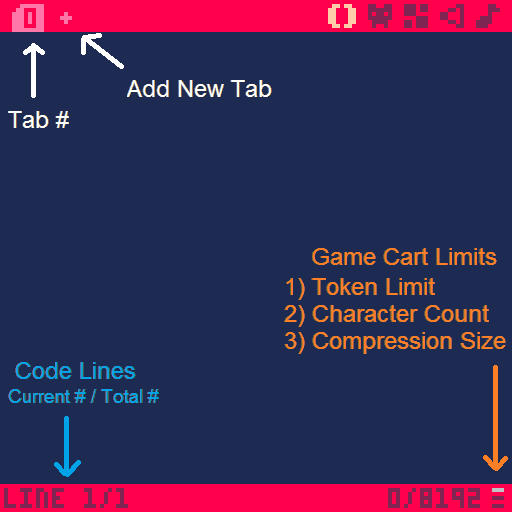• ### Sprite Editor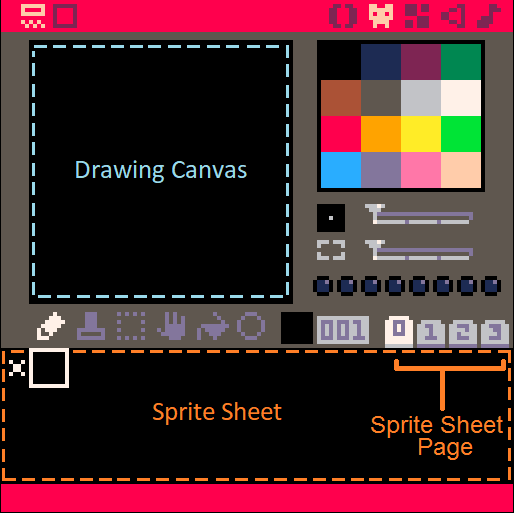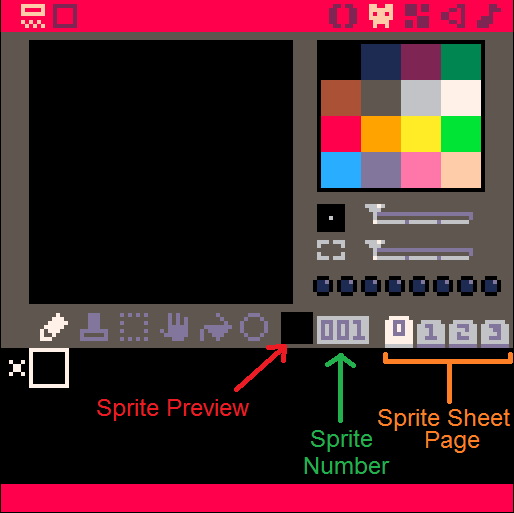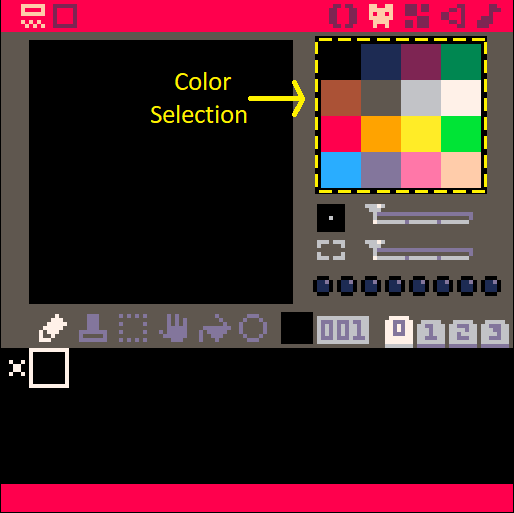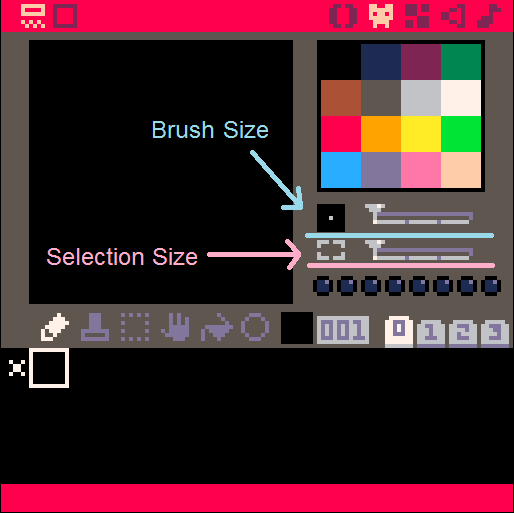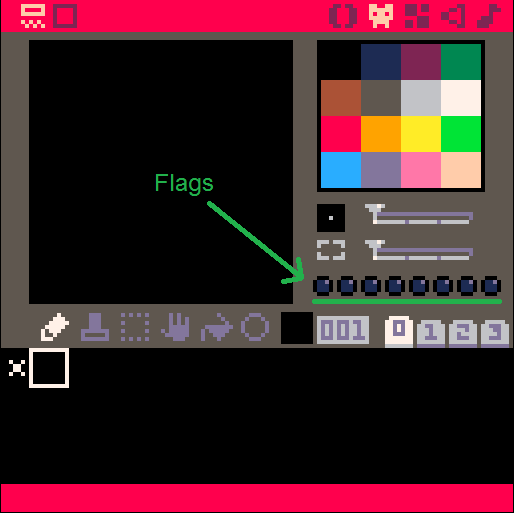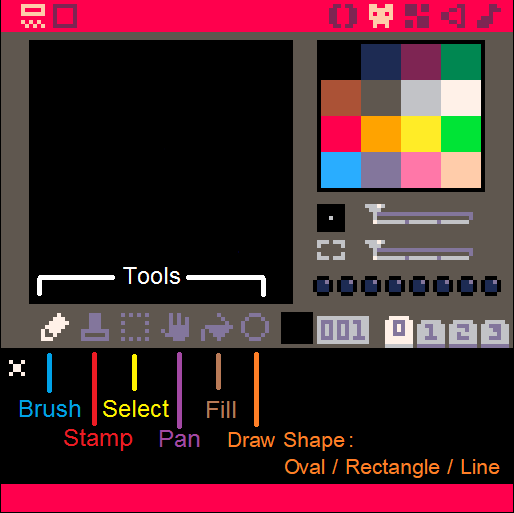• ### Map Editor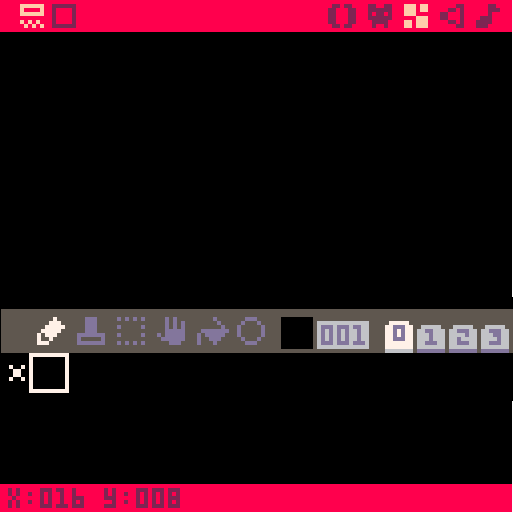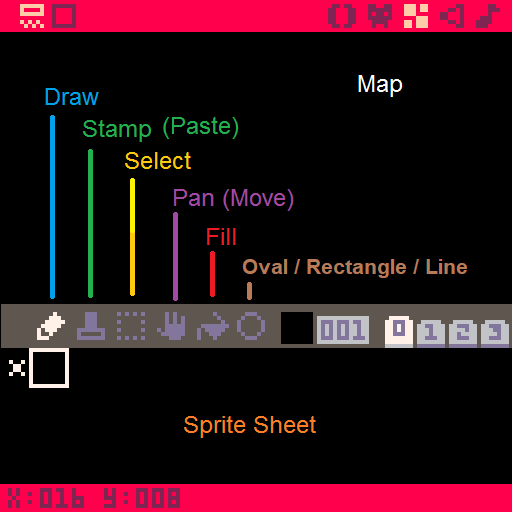• ### Sound Effects (SFX) Editor

Pitch Mode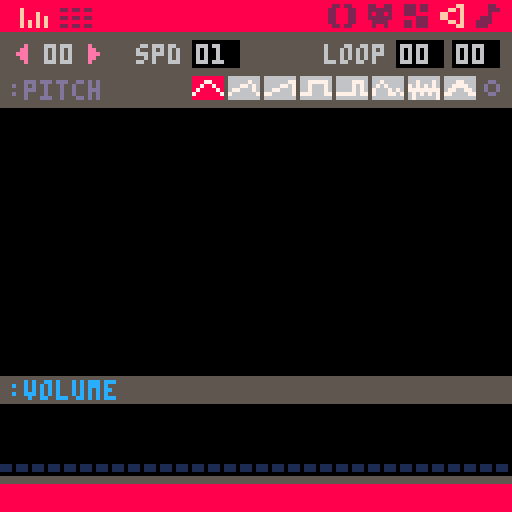Instruments and Loop Controls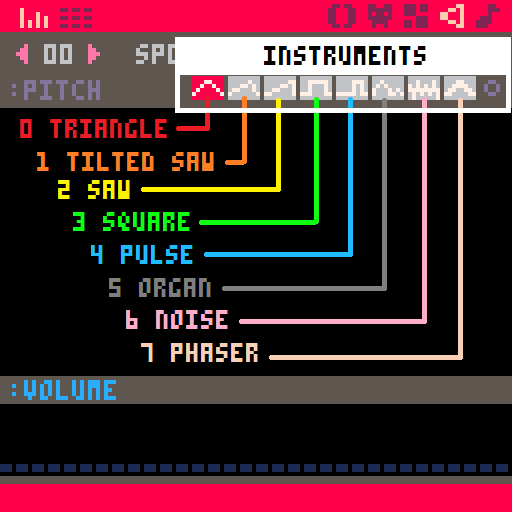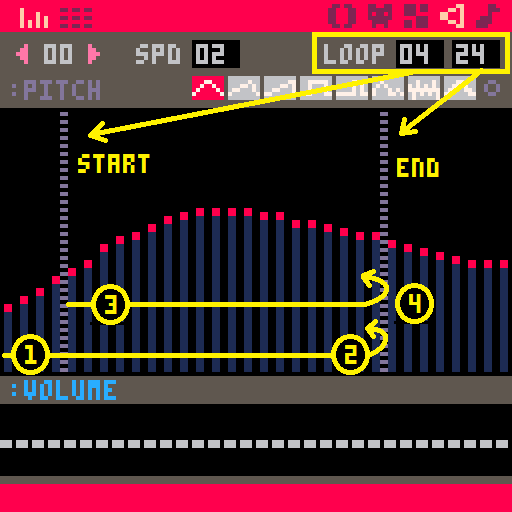Tracker Mode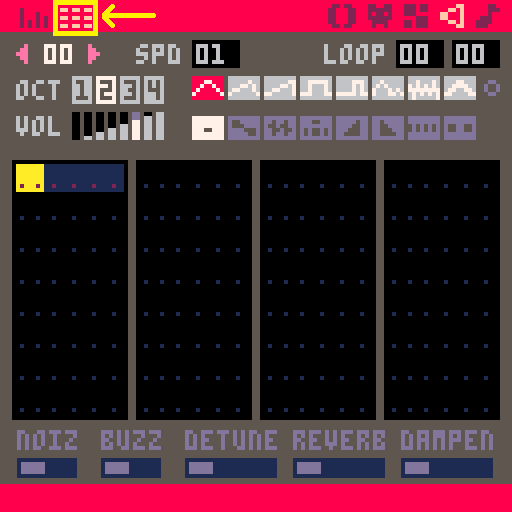• ### Music Editor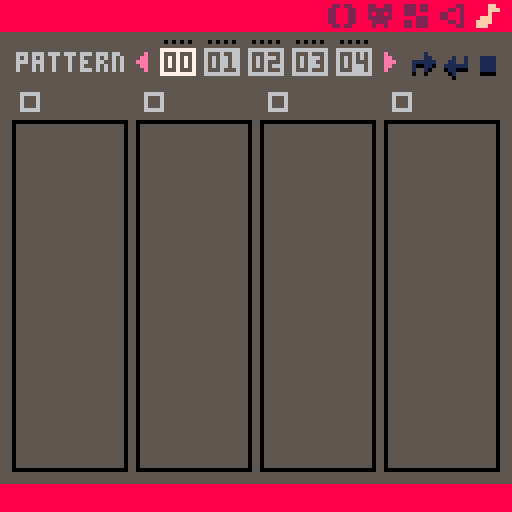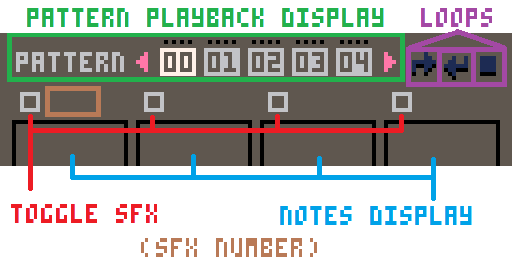Example of a selected SFX (#1) being used in a Music Pattern: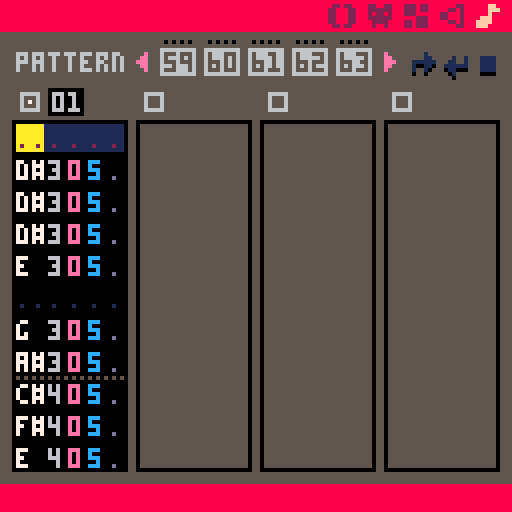• ## PICO-8 Commands

• ### Get Help

`help`

lists all commands to navigate PICO-8

• ### See Files and Folders

`dir` or `ls`

dir = "directory" (folder)

ls = "list"

shows all files and folders inside the folder currently open

• ### Open Folder outside of PICO-8

`folder`

Opens the current folder in a new window, outside of PICO-8

View the actual saved PICO-8 files and folders.

• ### Open Folder inside PICO-8

`cd folder_name`

cd = "change directory" or "change folder"

Opens the folder with that name if it is inside the folder already open

• ### Make Folder

`mk dir folder_name`

mk = "make"

dir = "directory" (folder)

creates a new folder inside the folder already open

• ### Back 1 Folder

`cd ..`

cd = "change directory" or "change folder"

.. = "back"

steps back to the parent folder that holds the folder currently open

`splore`

splore = "explore"

`load game_name`

loads the game if that game file is in the folder already open

• ### Save Game

`save game_name`

or anywhere in PICO-8, press: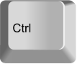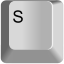• ### Play Game

`run`

Type this into the command screen.

run = play the loaded game

or anywhere in PICO-8, press: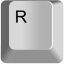• ### Exit Gameexits the running game

• ### Continue Game

`resume`

Resume = "continue"

resumes the running game from last exit point

• ### Edit GameOpens game editor of the loaded game

If game is running, press ESC twice

• ### Set Player Buttons

`keyconfig`

"keyconfig" = key configuration (button set up)

Opens button editor

• ### Restart PICO-8

`reboot`

Reboot = "restart"

restarts the whole PICO-8 system, make sure you save first!

• ### Exit PICO-8

`shutdown`

closes the whole PICO-8 system, make sure you save first!

• ## Getting Started Coding

• ### Set up the Game

``function _init()``

init = "initiate"

do something one time when game starts

not needed for the game to run

• ### Update the Game

``function _update()``

do something every frame we see: 30 times per second

Frame: 1 picture on screen.

Needed for game to run.

``function _update60()``

or use this for 60 times per second

• ### Draw to the Game Screen

``function _draw()``

do something every frame we see: 30 times per second

Frame: 1 picture on screen

Needed for game to run

• ## Screen

• ### Write on Screen

`` print( string, x, y, color)``

print = write on the screen at (x, y) position, in a color

x = (optional) number of pixels from the left going right

y = (optional) number of pixels from the top going down

color = (optional) a color number

• ### Set Position on Screen

`` cursor( x, y )``

cursor = imagine a mouse pointer on the screen(PICO-8 games do not have a mouse)

x = number of pixels from the top left corner going right

y = number of pixels from the top left corner going down

• ### Erase Screen

`` cls()``

CLS = "clear screen"

erases the entire screen

Erasing and re-drawing everything on the screen every frame is standard practice. So you will almost always want to have this in the first line of the `_draw()` function.

• ### Move camera

`` camera( x, y )``

sets the camera to position on screen

x = number of pixels from the top left corner going right

y = number of pixels from the top left corner going down

`` camera()``

reset camera to 0,0 position

• ### Crop the screen

`` clip( x, y, w, h )``

Clipping is for creating a smaller area to draw in.

x = number of pixels from the top left corner going right

y = number of pixels from the top left corner going down

w = width of clipping rectangle in pixels

h = height of clipping rectangle in pixels

After using this function, the screen will not draw outside of the clipped area.

``clip()``

Reset to fullscreen, no clipping

• ## Draw Shapes

• ### Circle Outline

`` circ( x, y, radius, color )``

circ = circle

x = number of pixels from the top left corner going right

y = number of pixels from the top left corner going down

x,y = the position of the center of the circle

radius = number of pixels from the center to the outside

color = (optional) color number

• ### Circle Filled

`` circfill( x, y, radius, color )``

circfill = circle filled

x = number of pixels from the top left corner going right

y = number of pixels from the top left corner going down

x,y = the position of the center of the circle

radius = number of pixels from the center to the outside

color = (optional) a color number

• ### Line

`` line(x1, y1, x2, y2, color)``

circ = circle

x = number of pixels from the top left corner going right

y = number of pixels from the top left corner going down

x1, y1 = the position of the start of the line

x2, y2 = the position of the end of the line

color = (optional) a color number

• ### Rectangle Outline

`` rect( x1, y1, x2, y2, color )``

rect = rectangle

x = number of pixels from the top left corner going right

y = number of pixels from the top left corner going down

x1, y1 = the position of the first corner

x2, y2 = the position of the opposite corner

color = (optional) a color number

• ### Rectangle Filled

`` rectfill( x1, y1, x2, y2, color )``

rectfill = rectangle filled

x = number of pixels from the top left corner going right

y = number of pixels from the top left corner going down

x1, y1 = the position of the first corner

x2, y2 = the position of the opposite corner

color = (optional) a color number

• ### Draw Patterns

`` fillp( glyph )``

fillp = fill pattern

glyph = one of the special PICO-8 font characters that replace capital letters.

• ### Write Glyphs

`` print( glyph ) --as a string``
or
`` fillp( glyph )``

glyph = one of the special PICO-8 font characters that replace capital letters.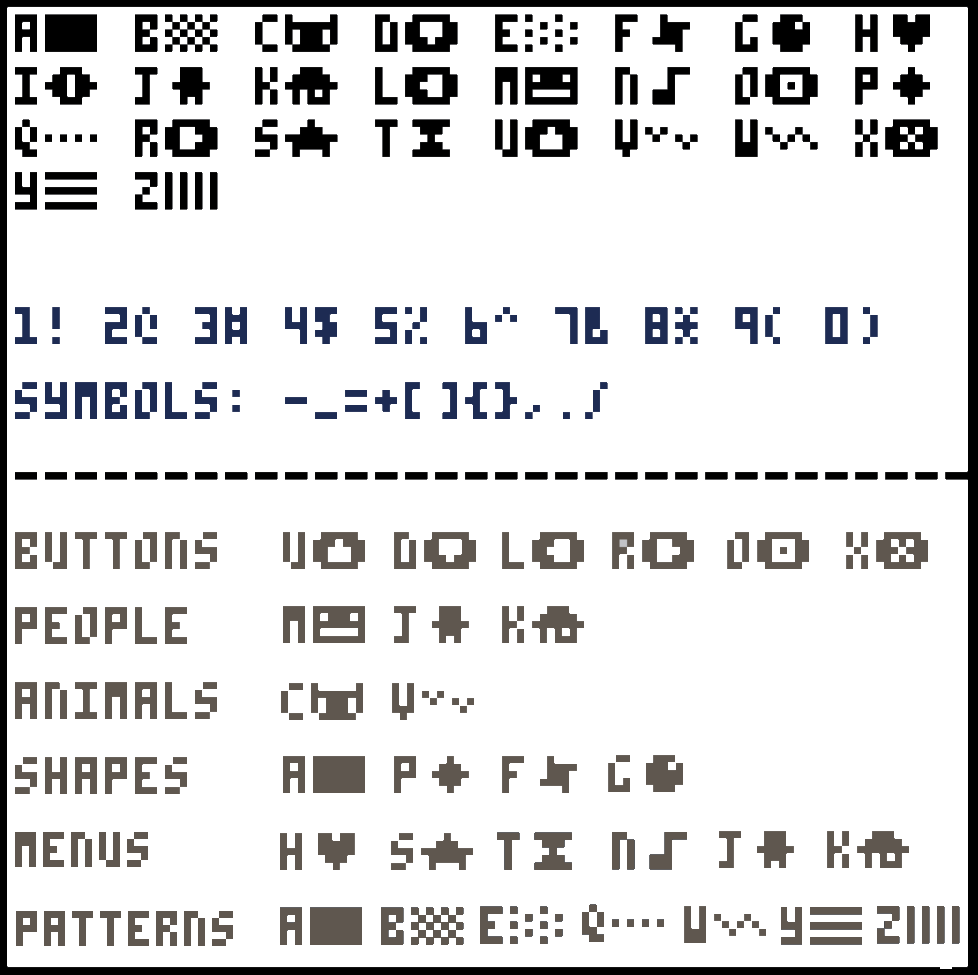• ## Colors

• ### Color Numbers

`00` (#000000) black
`01` (#1D2B53) navy
`02` (#7E2553) maroon
`03` (#008751) emerald
`04` (#AB5236) brown
`05` (#5F574F) charcoal
`06` (#C2C3C7) gray
`07` (#FFF1E8) white
`08` (#FF004D) red
`09` (#FFA300) orange
`10` (#FFFF27) yellow
`11` (#00E756) green
`12` (#29ADFF) sky
`13` (#83769C) lavender
`14` (#FF77A8) pink
`15` (#FFCCAA) peach
`128` (#291814) onyx
`129` (#111D35) midnight
`130` (#422136) plum
`131` (#125359) forest
`132` (#742F29) chocolate
`133` (#49333B) eggplant
`134` (#A28879) beige
`135` (#F3EF7D) lemon
`136` (#BE1250) burgundy
`137` (#FF6C24) pumpkin
`138` (#A8E72E) lime
`139` (#00B543) jade
`140` (#065AB5) blue
`141` (#754665) mauve
`142` (#FF6E59) coral
`143` (#FF9D81) salmon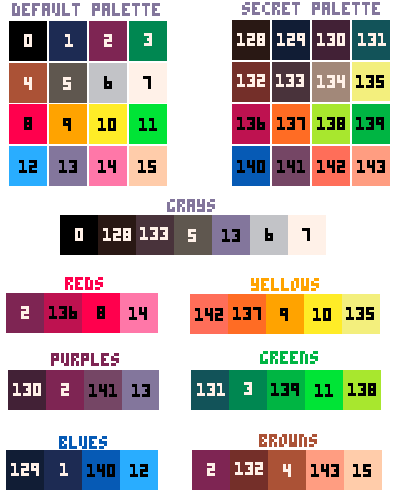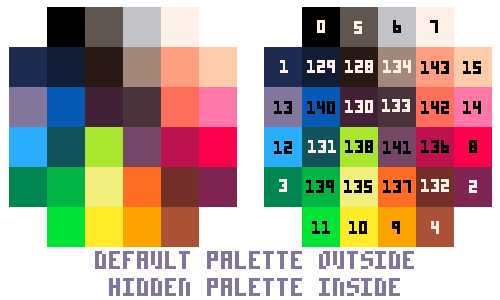• ### Select Color

`` color( number )``

select the color to be used

This will set the color to be used in the next use, such as: `print() circ() rect() line()`

If those and functions like them do not use the optional color setting, then whatever color was last set will be used. By using the optional color settings in functions that have them, it sets the new color to be the currently selected color.

Example:

``````color(8)
--changed color to red
print("hello world")
--hello world will be in red``````

The above is the same as:

``````print("hello world",0,0,8)    --0 for x, 0 for y, 8 for red
--hello world will be in red``````

This is how they work together:

``````print("hello",0,0,8)    --0 for x, 0 for y, 8 for red
--hello will print in red
color(7)
--changed color to white
print("world")
--world will print in white
``````

• ### Get Color from Screen

`` pget( x, y )``

`pget` = "pixel get" (1 pixel)

x = number of pixels from the top left corner going right

y = number of pixels from the top left corner going down

Returns the color number of the pixel at location (x,y) on the screen.

• ### Set Color to Screen

`` pset( x, y, color )``

`pset` = "pixel set" (1 pixel)

x = number of pixels from the top left corner going right

y = number of pixels from the top left corner going down

color = (optional) a color number

• ### Get Color from Sprite Sheet

`` sget( x, y )``

`sget` = "sprite sheet get" (1 pixel)

x = number of pixels from the top left corner going right

y = number of pixels from the top left corner going down

Returns the color number of the pixel at location (x,y) on the sprite sheet (not screen).

Both x and y must be within the range of 0 and 127.

• ### Set Color to Sprite Sheet

`` sset( x, y, color )``

`sset` = "sprite set" (1 pixel)

x = number of pixels from the top left corner going right

y = number of pixels from the top left corner going down

color = (optional) a color number

• ### Swap Colors

`` pal( current color, new color, boolean )``

`pal` = "palette" (set of colors)

current color = color number to change

new color = color number to change to

boolean = (optional) 0 for next draw or 1 for already drawn, default 0.

`` pal()``

resets all colors to the default palette

`` pal(table)``

swap full palette using a table.

`` pal({1,2,3,...})``

This will swap color numbers 1-15 (in order) to the numbers set within the table.

• ### Make Color Transparent

`` palt( color, boolean )``

`palt` = "palette transparent"

color = a color number

boolean = true or false to set transparency on or off

true = do not draw this color pixel

false = do draw this color pixel

`` palt()``

resets all colors to default transparency, where only black (#0) is transparent

• ### Hidden Colors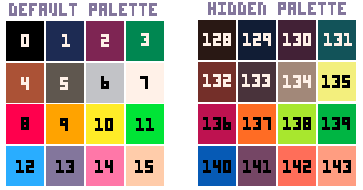PICO-8 has 16 extra colors that are undocumented and a bit tricky to access.

This means we actually have 32 colors to choose from and you can set your own 16 color palette using a mix of them both.

`` pal( current color, new color, 1 )``

Using the palette swapping function, you can change a default color with a hidden color but you must set the last argument to 1.

``poke(0x5f2e,1)``

This accesses the PICO-8 memory and sets the color palette to change. Do this to see the code below.

`` pal({1,2,3...},1)``

swap the full palette using a mix of default and hidden color numbers in a table and the second argument set to 1.

`` pal({=128,129,130,131,132,133,134,135,136,137,138,139,140,141,142,143},1)``

swap all of the default colors to the hidden colors.

• ## Sprites

• ### What is a Sprite?

sprite = a drawing using multiple pixels that can be considered as a single thing.

A character, item, or piece of the background are usually made of one or many sprites. Multiple sprites that look similar but have small changes between them are often used to create sprite animations.

A sprite has a width (W) and a height (H). The W is the number of pixels wide and the H is the number of pixels tall.
We can communicate the size of a sprite by saying these two numbers together.
The size of a sprite is called its "dimensions".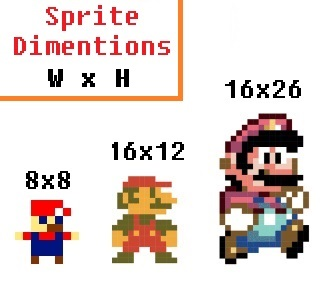As you can see, the bigger sprite looks better because it has more pixels and can show more detail. In fact, even the most beautiful and detailed digital pictures are just made of many many pixels. Here is how we can take the same character and draw him in larger dimensions in PICO-8.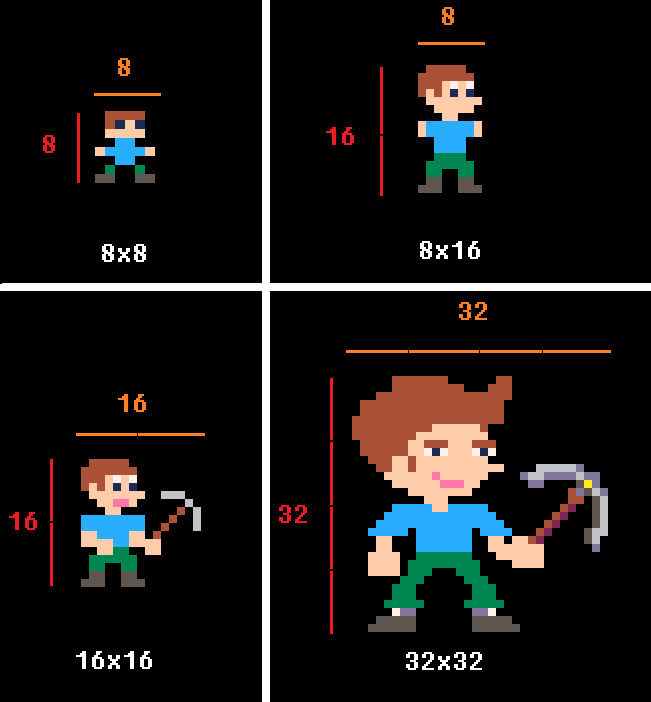• ### What is a Sprite Sheet?

sprite sheet = a collection of multiple sprites.

You can find the PICO-8 sprite sheet in the sprite editor. Each PICO-8 game has one sprite sheet that appears broken up into 4 pages. The sprite editor is more than just a place to draw our sprites:Example of a full sprite sheet (1st page):Here, you can see multiple sprites in a sprite sheet used to create movement animation.
Each small monkey is a single tile (8x8) and there are 9 different sprites that are used to make it look like one monkey is running in the game.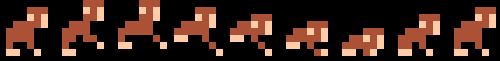Hover your mouse over a selected sprite's top left corner and you will see the x and y values of the sprite location on the sprite sheet. This is useful for the SSPR( ) function.

• ### Draw a Sprite on Screen

``spr( sprite number, x, y, w, h, flip x, flip y )``

#### SPR( ) Full Tutorial!

spr = "sprite"

sprite # = a number of the sprite in the sprite sheet

x = a number of pixels from the top left corner of the game screen going right

y = a number of pixels from the top left corner of the game screen going down

w = (optional) a number for how wide the sprite is in tiles (8 pixels in 1 tile) Default = 1

h = (optional) a number how tall the sprite is in tiles (8 pixels in 1 tile) Default = 1

flip x = (optional) true or false for flipping or reversing the sprite from left to right / right to left

flip y = (optional) true or false for flipping or reversing the sprite from top to bottom / bottom to top

If you do not include columns and rows, the default is 1. So the simplest form of this code draws an 8x8 sprite.

Example of a 16x16 pixel sprite: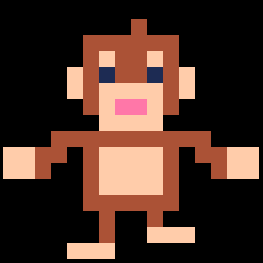A sprite is always drawn starting from the top left corner of the sprite. So the X and Y coordinates given will be the location for the top left pixel of the sprite.

If you flip the sprite on the X or Y, this only changes the way it is drawn, not the position on the screen.

• ### Draw a Sprite of Any Size

``sspr( sx, sy, w, h, x, y, dw, dh, flip x, flip y )``

`sspr` = "special sprite" ?

sx = "sprite x" number of pixels right in sprite sheet to the top left corner of the sprite

sy = "sprite y" number of pixels down in sprite sheet to the top left corner of the sprite

w = "width" number of pixels wide from sx

h = "height" number of pixels high from sy

x = # pixels from the left to start drawing sprite on screen

y = # pixels from the top to start drawing sprite on screen

dw = (optional) the width of the sprite drawn on the screen. Default = w

dh = (optional) the height of the sprite drawn on the screen. Default = h

flip x = (optional) If true, the image is drawn inverted left to right. Default = false

flip y = (optional) If true, the image is drawn inverted top to bottom. Default = false

A sprite is always drawn starting from the top left corner of the sprite. So the X and Y coordinates given will be the location for the top left pixel of the sprite.

If you flip the sprite on the X or Y, this only changes the way it is drawn, not the position on the screen.

• ## Maps

• ### Draw Part of Map on Screen

``map( tile x, tile y, x, y, w, h, flag )``

tile x = column number of top left corner of map section in tiles (8 pixels per tile)

tile y = row number of top left corner of map section in tiles (8 pixels per tile)

x = # pixels from the left to start drawing map section on screen

y = # pixels from the top to start drawing map section on screen

flag = (optional) a flag number to specify certain sprites.

• ### Get Sprite on Map

``mget( column, row )``

`mget` = "map get"

columns = number of tiles from the top (8 pixels in 1 tile)

rows = number of tiles from the right (8 pixels in 1 tile)

Example:
``````player_column = player_x / 8
player_row = player_y / 8

mget( player_column + 1, player_row )``````

This is useful for finding certian things on the map near the player. In the code above, you get what sprite number is to the right of the player on the map. For example, I might want to check for a door on the map.

• ### Set Sprite on Map

``mset( column, row, sprite number )``

`mset` = "map set"

columns = number of tiles from the top (8 pixels in 1 tile)

rows = number of tiles from the right (8 pixels in 1 tile)

sprite number = number of the sprite in the sprite sheet

Example:
``````playerColumn = playerX / 8
player_row = playerY / 8
door_column = 4
door_row = 5
door_open = 20 --sprite #
door_close = 21 --sprite #

if player_column == door_column
and player_row == door_row then
mset( door_column, door_row, door_open )
else
mset( door_column, door_row, door_close )
end``````

This is useful for changing certain things on the map, when something happens. In the code above, we check if the player is standing on a door, then set the door sprite to be open, else set it closed.

• ## Strings and Variables

• ### What is a String?

``"any text inside quotation marks"``

A string is a line of text. It can be one letter or a full sentence, or just symbols.

This tells the computer to see anything inside quotation marks (" ") as just letters and symbols, not as numbers or variables.

Example:
``````b = "hello"
print( b ) --prints: hello``````

Without quotation marks, it is a variable name. Example error:

``````a = hello
print( a ) --prints [nil]``````

nil (null, nothing) printed because the computer was looking for a variable named `hello`, not a string.

• ### Count the Length of the String

``#string``

Placing a # in front of a variable name that holds a string counts how many characters are in the string.

Example:
``````a = "hello"
print( #a ) -- prints: 5``````

• ### What is a Variable?

``variable = "information"``

A variable is a letter, symbol, or word that holds information.

This tells the computer to make the variable hold the information and remember them.

Example 1:
``````a = 1
print( a ) --prints: 1``````

Anytime the Computer sees A, it will remember that A is 1.

Example 2:
``````b = 20
c = 10
print( b + c ) --prints: 30``````

The computer understands B as 20 and C as 10 so adding B and C, the computer adds 20 and 10 and prints the answer.

A variable can hold a "string": `words = "these are words"`

A variable can hold a "numeral": `num = 120`

A variable can hold a "boolean": `bool = true`

A variable can hold a "table": `tbl = { key = value }`

A variable can hold a "function": `p = print`

(This is specific to the Lua programming language)

• ### Mix Strings with Variables

``string..variable``

the .. tells the computer to read a string and then change to read a variable, or the other way around.

Example:
``````points = 10
print( "score= "..points ) --prints: score= 10``````

• ## Tables

• ### What is a table?

Table = a set of information placed into rows and columns

You can collect and organize your game information easily into tables.

You can't see the tables in PICO-8, so you will have to imagine them.

Example:
 Column 1(key) Column 2(value) 1 "A" 2 "B" 3 "C"

Key = The first column's data. A number or string used to find the data stored in Column 2

Value = The second column's data. A number, string, boolean, or table that you want to store

• ### Create an Empty Table

``variable = { }``

{ = starts the table

} = ends the table

Example:
``fruit = { }``
 Fruit

• ### Create a Table as a Simple List

``variable = { a, b, c }``

{ = starts the table

} = ends the table

a, b, c, = data added to the table and automatically placed in rows 1, 2, and 3

Example:
``colors = { "red", "blue", "pink", "yellow" }``
 Colors 1 "red" 2 "blue" 3 "pink" 4 "yellow"

• ### Create a Table with Named Keys

``variable = { a=b, c=d, e=f }``

{ = starts the table

} = ends the table

a=b = information added to the table and put in key: A linked with value: B

Example:
``highscores={ "john"=10, "bob"=143, "kim"=984 }``

Or write it like this:

``````highscores = { }      --creates empty table
highscores.john = 10 -- adds key "john" with value 10
highscores.bob = 143
highscores.kim = 984``````

This is what it would look like:

 HighScores john 10 bob 143 kim 984

• ### Create a Table inside another Table

``variable = { a={ }, b={ } }``

{ = starts the table

} = ends the table

a={ } : First table (named a) inside main table

b={ } : Second table (named b) inside main table

Example of Named Keys:
``````items={
sword={ name="iron sword", strength=20 },
shield={ name="wood shield", strength=8 }
}``````
Items
sword  name iron sword strength 3
shield  name wood shield strength 8

You may also insert tables without key names, so that each item added is automatically numbered.

Example of Numbered Keys:
``````enemies = {
{ health=10, attack=3 },
{ health=20, attack=8 }
}``````
Enemies
1  health 10 attack 3
2  health 20 attack 8

• ### Add to a Table

``add( table, value )``

table = the variable name of the table

value = the information to add to the table

, = commas separates the table name with the information to add

Example:
``colors = { "red", "blue", "pink", "yellow" }``
 Colors 1 "red" 2 "blue" 3 "pink" 4 "yellow"
``add( colors, "purple" )``
 Colors 1 "red" 2 "blue" 3 "pink" 4 "yellow" 5 "purple"

• ### Delete from a Table

``del( table, value )``

table = the variable name of the table

value = the data to delete from the table

, = commas separates the table name with the data to delete

Example:
 Colors 1 "red" 2 "blue" 3 "pink" 4 "yellow"
``del( colors, "blue" )``
 colors 1 "red" 2 "pink" 3 "yellow"

Notice that BLUE was key #2. Deleting BLUE, also moved the rest of the list up one number.

This happens for tables that have numbered keys, but it does not change tables that have keys that are strings.

• ### Get the Total Number of things in a Table

``#table``

# = count the number of items in a table

Example:
 HighScores john 10 bob 143 kim 984

``#highscores --count: 3``

• ### Set Value in a Table

``table[ key ] = value``

[ ] = the key of the table(left column)

Example:
 HighScores john 10 bob 143

``highscores[ "john" ] = 30``
 HighScores john 30 bob 143

Or:

``table[ number ] = value``

[ ] = the key of the table(left column)

Example:
 Colors 1 ""red" 2 "blue" 3 "pink"

``colors[ 2 ] = "orange"``
 colors 1 "red" 2 "orange" 3 "pink"

• ### Get Value from a Table with Numbered Keys

``table[ number ]``

[#] = the number key of the table (left column)

Example:
 Colors 1 "red" 2 "orange" 3 "pink"

``print( colors[ 2 ] ) --prints: orange``

• ### Get Value from a Table with Named Keys

``table[ key ]``

OR

``table.key``

[ ] = the variable name key of the table (left column)

This is just like a variable, so just asking for the table and key, it will be whatever the value is in the table at that key.

Example:
 HighScores john 10 bob 143

``print( highscores[ "john" ] ) -- prints: 10``
``print( highscores.john ) -- prints: 10``

• ## Loops

• ### Repeat a Certain Number of Times

``````for variable = start, stop, count_by do
--code to repeat
end``````

variable = a letter or word to set as the current repeat count

start = a number for the repeat count to start at

stop = a number for the repeat count to end at

count by = a number for the count to increase or decrease by (optional, default 1) To count down, make this a negative number.

"FOR LOOPS" begin counting from the "start" number, count up or down by the "count by" number, and stops at the "stop" number.

Example:
``````for i = 1, 9, 2 do
print( i )
end

--prints: 1 3 5 7 9``````

If the "count by" number is left out, then it will automatically be 1.

Example:
``````for i = 1, 9 do
print( i )
end

--prints: 1 2 3 4 5 6 7 8 9``````

If you want to start at a high number and count down, then make the "count by" number a negative number.

Example:
``````for i = 10, 3, -1 do
print( i )
end

--prints: 10 9 8 7 6 5 4 3``````

• ### Repeat Until something Happens

``repeat code until condition ``

code = whatever code you want to repeat

condition = a variable or statement that will resolve to true or false

"REPEAT LOOPS" continue doing the code after REPEAT and stops repeating when the condition is true.

Example:
``````a = 0
repeat
print( a )
a += 1
until a == 5

--prints: 0 1 2 3 4``````

NOTE: Repeat always runs the code at least once, before checking the conditional.

• ### Repeat While something is Happening

``````while condition do
code
end``````

condition = a variable or statement that will resolve to true or false

code = whatever code you want to repeat

"WHILE LOOPS" repeat the code after DO and stops repeating when the condition is false.

Example:
``````b = 4
while b < 10 do
print( b )
b += 1
end

--prints: 4 5 6 7 8 9``````

• ### Repeat this For Each Entry in a Table

``foreach( table, funct )``

table = a variable holding a table

funct = name of a function to run multiple times

Example:
 HighScores john 10 bob 143 kim 984

``foreach( highscores, sort )``

From the first to the last entry in HIGHSCORES table, send each through the SORT function.

FOREACH passes the table to the function so SORT function should be ready to receive a table as a variable like this: `function sort( table )`.

• ### Repeat for All Entries in a Table

``for variable in all( table ) do``

new variable = a local variable name that will hold the value of each entry from the table

table = a variable that holds a table

Example:
 HighScores john 10 bob 143 kim 984
``````for score in all( highscores )
print( score )
end

-- prints: 10 143 984``````

To get both the value and the key from a table, use:

``for key, value in pairs( table ) do``

key = any variable name to set as the table item in the left column

value = any variable name to set as the table item in the right column

table = name of table

``````for name, score in pairs( highscores )
print( name )
print( score )
end

-- prints: john 10 bob 143 kim 984``````

• ### Force a Loop to Stop

``break``
Example:
``````for i = 0, 10 do
if i == 5 then
break
end
print ( i )
end

--prints 0 1 2 3 4
``````

• ## Save and Export

• ### Save Game

`save gamename`

Type this into the command screen of PICO-8.

Or, anywhere in PICO-8, do this:• ### Save Game Label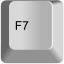This takes a screen shot of your game, and saves it as the game's label when creating the game cartridge.

• ### Save Game Cartridge

`save gamename.p8.png`

Type this into the command screen of PICO-8.

This saves your game cartridge as an image, with the label on the cover.

You can share this image with others who have PICO-8 and they can play it in PICO-8.

WAIT! Before you save your game image, you should write this in the first two lines of your game's code and it will show on your game cartridge:

``````--(game name)

Example:• ### Export Game as Website

`export gamename.html`

Type this into the command screen of PICO-8.

This exports the game for websites. It gives you two files that need to stay together. One is .html and the other is .js

• ### Export Game as Desktop App

`export gamename.bin`

Type this into the command screen of PICO-8.

This exports the game as a standalone application. Share your game with anyone! They don't need PICO-8 to play it.

• ### Export Sprite Sheet

`export gamename.png`

Type this into the command screen of PICO-8.

This exports the sprite sheet as an image in png format.

• ### Export Sound FX

`export gamename.wav`

Type this into the command screen of PICO-8.

This exports the game's sound effects as a wav file. Share your game with anyone! They don't need PICO-8 to play it.

• ## Controls (Inputs)

• ### Check Button Press

``btn( number , number )``

First Number = a button number or button symbol (see chart below)

Second Number = (optional) a player number (0-7)

If you hold the button down, this will act like it is rapidly pressed.

``btnp( number , number )``

First Number = a button number or button symbol (see chart below)

Second Number = (optional) a player number (0-7)

If you hold the button down, this limits one press every other game frame.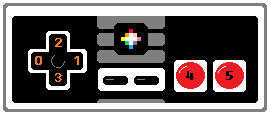For multiplayer games, you will need to include player numbers: 0-7

Instead of button numbers, you can also use the symbols.

 Button Number Symbol Left 0 shift+L Right 1 shift+R Up 2 shift+U Down 3 shift+D O 4 shift+O X 5 shift+X

• ## Music and Sound Effects (SFX)

• ### Play a Sound Effect

``sfx( sfx-number, channel, offset )``

sfx-number = the SFX number of the sound you want to play or stop

channel = (optional) a number specifying which of the 4 channels (#0-3) to play on.

offset = (optional) a number of notes into a sound for when to start playing the sound

If sfx-number is -1, current sound on the channel is stopped

If sfx-number is -2, it will finish the sound but stop looping.

If channel is -1 (default), a channel that is not in use will be picked automatically.

Example:

``sfx( 1 ) -- play SFX-number 1``
``sfx( 3, 0 ) -- play SFX-number 3 in channel 0``
``sfx( 4, 3, 6 ) -- play SFX-number 4 in channel 3, start 6 notes in``

• ### Stop a Sound Effect

``sfx( -1, channel)``

channel = (optional) a number specifying which of the 4 channels (#0-3) to play on.

• ### Play Music

``music( music-number, channel, offset )``

music-number = the number of the song in the music editor.

channel = (optional) a number specifying which of the 4 channels (#0-3) to play on.

offset = (optional) a number of notes into the music for when to start playing the music

Example:

``music( 1 ) -- play music number 1``
``music( 3, 0 ) -- play music 3 in channel 0``
``music( 4, 3, 8 ) -- music 4, channel 3, start 8 notes in``

• ## Math

• ### Round a number down

``flr( number )``

`flr` = ("floor") find the nearest whole number by rounding down

Number = any number or decimal

Example:

``a = flr( 1.6 ) -- a = 1``
``b = flr( 7 / 3 ) -- b = 2``

• ### Make a number Positive

``abs( number )``

`abs` = ("absolute") same number but ignore the sign( + / - )

Number = any number or decimal

Example:

``a = abs( 16 ) -- a = 16``
``b = abs( -2.5 ) -- b = 2.5``
``d = abs( 1.5 - 2 ) -- d = 0.5``

• ### Get a number's sign: ( + / - )

``sgn( number )``

`sgn` = "sign" positive or negative ( + / - )

Number = any number or decimal

If # is positive, SGN(#) = 1

If # is zero, SGN(#) = 1

If # is negative, SGN(#) = -1

Example:

``a = sgn( 16 ) -- a = 1``
``b = sgn( 0 ) -- b = 1``
``c = sgn( -2.5 ) -- c = -1``
``d = sgn( 5 - 16 ) -- d = -1``

Notice that SGN( 0 ) returns 1, simply as a non-negative number.

This is a handy function for recognizing 0 and returning: ( 1 / 0 / -1 )

``````function truesgn(a)
local r=0
if (a!=0) r=sgn(a)
return r
end``````

(shared by @dw817)

• ### Square Root

``sqrt( number )``

`sqrt` = "square root"

Number = any number or decimal

What is Square Root?

To square a number, means you times the number by itself:
3 x 3 = 9 or "3-squared" = 9

Square Root is the opposite and finds what number times itself equals the #:
"square-root of 9" = 3

Example:

``a = sqrt( 16 ) -- a = 4``
``b = sqrt( 100 ) -- b = 10``
``c = sqrt( 30 - 21 ) -- c = 3 ``

• ### Get Larger Number

``max( number , number )``

`max` = "maximum"

First Number = any number or decimal

Second Number = (optional) any number or decimal, defaults to 0

This compares two numbers and returns the higher value

Example:
``a = max( 16 , 25 ) -- a = 25``
``a = max( 5 ) -- a = 5``
``a = max( -10 ) -- a = 0``
``b = max( 20+30 , 100-30 ) -- b = 70``

• ### Get Smaller Number

``min( number , number )``

`min` = "minimum"

First Number = any number or decimal

Second Number = (optional) any number or decimal, defaults to 0

This compares two numbers and returns the lower value.

Example:
``a = min( 16 , 25 ) -- a = 16``
``a = max( 5 ) -- a = 5``
``a = max( -10 ) -- a = 0``
``b = min( 20+30 , 100-30 ) -- b = 50``

• ### Get Middle Number

``mid( number , number , number )``

MID = "middle"

Number = any number or decimal

This sorts a list of numbers then gives the middle number ("median") of the list.

Example:
``a = mid( 25 , 10 , 40 ) -- a = 25``
``b = mid( 20+30 , 100-30 , 10+50 ) -- b = 60``

• ### Make a Wave

``sin( # ) -- sine wave``
``cos( # ) -- cosine wave``
``atan2( # , # ) -- arc tangent``
Examples:
Purple = Cosine
Green = Sine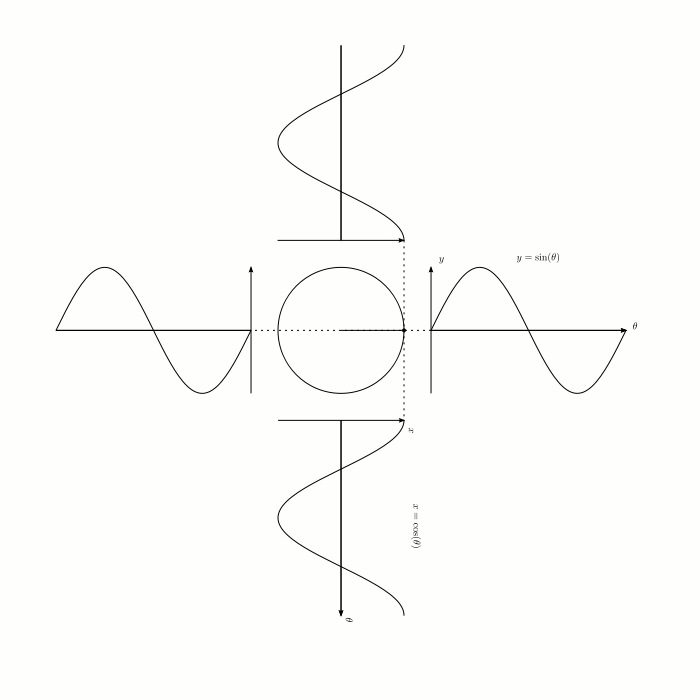• ### Random Number: Decimal

``rnd( number )``

`rnd` = "random"

Number = any number or decimal

Generates a random number from 0 to the number given (including 0 and number given)

Example:
``a = rnd( 8 ) --returns a decimal between 0 and 8``
``b = rnd( 20+30 ) --returns a decimal between 0 and 50``

• ### Random Number: Whole #

``flr( rnd( number ) )``

`rnd` = "random" (see Random Number: Decimal)

`flr` = "floor" (round down)

Number = any number or decimal

Generates a random number from 0 to the number given (including 0 and the number given) then rounds down to the nearest whole number and returns that.

Example:
``a = flr( rnd( 3 ) ) --gives whole number between 0 and 3``
``b = rnd( 5*10 ) --gives a decimal between 0 and 50``

This is a menu and coding reference for Pico-8.

`font`